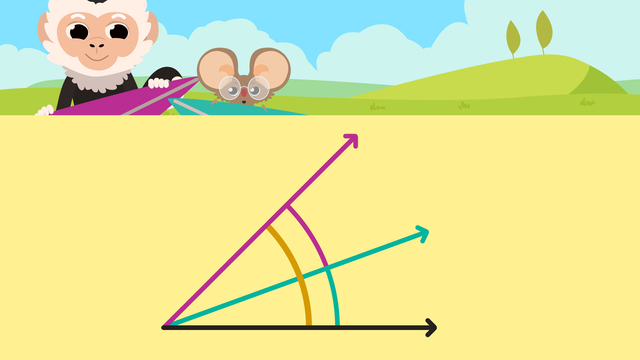Rating

Ø 5.0 / 3 ratings
The authorsTeam Digital
CCSS.MATH.CONTENT.4.MD.C.7

## Basics on the topicAdditive Angles

Did you know that you can add and subtract angles? Adding and subtracting angles happens, when an angle gets smaller or larger. If an angle gets larger, we speak of additive angles. Additive angles are made from adjacent angles. Additive means we can use addition or subtraction to solve for unknown angle measurements.

Adjacent angles are two angles that are next to each other but do not overlap. They share the same vertex and one side. Adjacent angles have smaller angles and also a larger one created when they are combined.The illustration below shows an example of how to add angles. The formula is rather simple. You add the adjacent angles to get a new whole.When we know the measurements of the parts of an adjacent angle, we can add the parts together to find the measurement of the total angle created.

part + part = whole
20° + 25° = 45°

If we add the two adjacent angles, we get a new whole of 45°.

Let’s look at an example of how to subtract angles. The illustration below shows an example of how to subtract angles. In order to subtract angles, you reverse the formula of how to add angles.When we know the measurement of the whole angle created by adjacent angles, we subtract the measurement of the known part from the total to find the missing measurement.

whole - part = part
140° - 30° = 110°

If we subtract 30° from our whole of 140°, we can conclude that our missing measurement is 110°!

## Adding and Subtracting Angles – Further Practice

Following the video, there is additional additive angles practice for 4th with interactive exercises and worksheet. The additive angles worksheet will have more examples of adjacent angles that have missing angle measurements.

Would you like to apply the knowledge you’ve learned? You can review and practice it with the tasks for the video Additive Angles .
• ### Testing the gliders.

Hints

Read the text to find the angles of Nico and Nia and where they can be marked on the image.

Find the largest angle by adding the two smaller angles together.

Solution

Nia's glider made an angle of 40°, this is shown in green. Nico's glider made and angle of a further 38°, this is shown in pink. When we add this on to Nia's angle: 40° + 38° = 78°. This is shown in orange.

• ### What is the angle of Nico's flight?

Hints

Since Nico flew 20° higher than Nia, we need to add 20° onto Nia's angle to find the angle of Nico's flight.

What is 20 + 35?

Solution
• To find the angle that Nico's glider flew from the ground, we need to add 20° to Nia's angle, since we know that Nico flew 20° higher than Nia.
• Nia flew 35° and Nico flew 20° higher.
• 35° + 20° = 55°.
• So Nico's glider flew 55° from the ground.
• ### Find the missing angle.

Hints

To find a missing angle when we know the total of 2 adjacent angles, take the known angle from the total.

What is the calculation that needs to be completed?

Solution
• Nico and Nia both make angles with their gliders, that total 75°.
• Since Nia's angle is 43°, to find Nico's angle we must subtract 43° from 75°.
• This will give us the missing angle.
• When we take 43° from 75° we get 32°, so this is the angle made by Nico.
• ### Missing angles.

Hints

Where two adjacent angles have been given, the total of these can be found by adding them together.

If one adjacent angle has been given, subtract this from the total to find the missing angle.

Look carefully where the question mark is to find the correct missing angle.

Remember that we can recognize a right angle as 90° because it is marked by a small square like this,

Solution

The solution image shows how the missing angle can be found for each question.

• ### Which image shows adjacent angles?

Hints

Adjacent angles share one straight line and a vertex.

Adjacent angles are next to each other. Remember, we are just looking at the angles marked in pink and green.

Solution
• The angles shown in a) are adjacent because they share a vertex and one straight line. This is the CORRECT answer.
For the other angles:
• The angles shown in b) do not share a vertex or straight line.
• The angles shown in c) share a vertex but do not share a straight line.
• The angles shown in d) share a vertex but do not share a straight line.
• ### Angles in a circle.

Hints

Look at the right angles marked in the circle, can this help you to find the missing angles?

Remember that there are 90° in a right angle and 180° on a straight line.

Solution
• To find angle a: We can see that there is a little square that means right angle, this means that the angle is 90°.
• To find angle b: The top right quarter of the circle is shown by a right angle, this is then split into two adjacent angles. One of these is given as 37°. To find the missing angle we need to subtract 37° from 90° to get 53°.
• To find angle c: There are two right angle symbols on the top half of the circle, so we know that the line going horizontally across the circle is a straight line and angles on a straight line must add up to 180°. As one of these adjacent angles is given here as 132° we then subtract 132° from 180° to get 48°.